# 葡萄糖在铜修饰碳糊电极上的电化学行为及测定Electrochemical Behavior and Determination of Glucose at Copper Modified Carbon Paste Electrode

DOI: 10.12677/HJCET.2019.91007, PDF, HTML, XML, 下载: 360  浏览: 868

Abstract: A copper modified carbon paste electrode was prepared by electrodeposition and the electro-chemical behavior of glucose at the modified electrode was investigated. The determination of glu-cose was achieved by chronoamperometry, revealing that the concentration of glucose changed linearly with the current when the concentration ranged from 1.01 × 10−7 to 1.20 × 10−5 mol/L, and the detection limit was 5.0 × 10−8 mol/L. The methodology was applied in detection of actual sam-ples, manifesting several features including high selectivity, quick analysis and easy operation.

1. 引言

2. 实验部分

2.1. 仪器与试剂

2.2. 修饰碳糊电极的制备

2.3. 实验方法

3. 结果与分析

3.1. 修饰电极的表征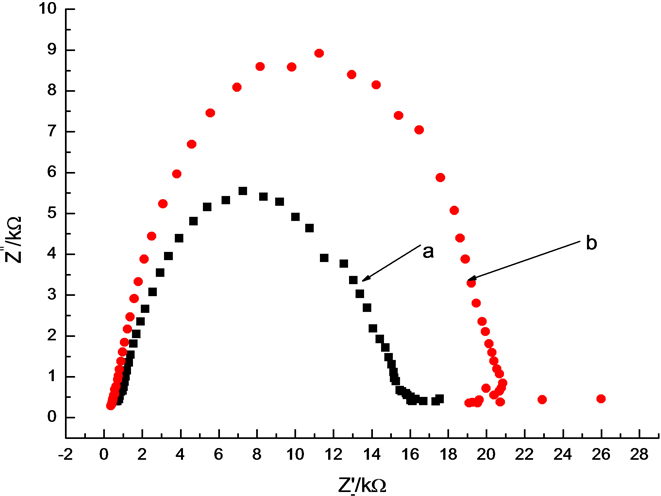Figure 1. Electrochemical impedance spectroscopy of bare carbon paste electrode (CPE) (b) and Cu modified carbon paste electrode (a)

3.2. 葡萄糖在修饰电极上的电化学响应

$\text{Cu}+2{\text{OH}}^{-}\to \text{CuO}+{\text{H}}_{2}\text{O}+2\text{e}$ (1)

$\text{CuO}+{\text{OH}}^{-}\to \text{CuOOH}\text{\hspace{0.17em}}或\text{\hspace{0.17em}}\text{CuO}+{\text{H}}_{\text{2}}\text{O}+{\text{2OH}}^{-}\to \text{Cu}{\left(\text{OH}\right)}_{4}^{-}+\text{e}$ (2)

$\text{CuOOH}+\text{e}+\text{Glucose}\to \text{Gluconolactone}+\text{CuO}+{\text{OH}}^{-}$ (3)

$\text{Gluconolactone}\to \text{Gluconic acid}$ (4)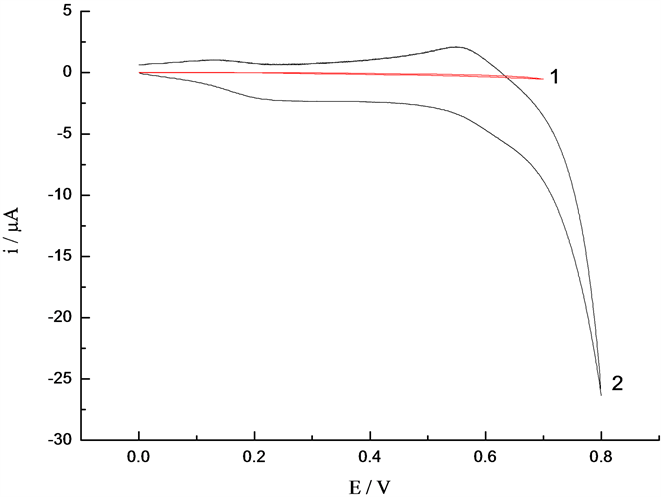Figure 2. Cyclic voltammograms of different electrodes by sweeping potential from 0 V to 0.8 V; 1: CPE, 2: CPE/Cu

3.3. 葡萄糖在不同修饰电极上的计时安培曲线

3.4. 电沉积电位的影响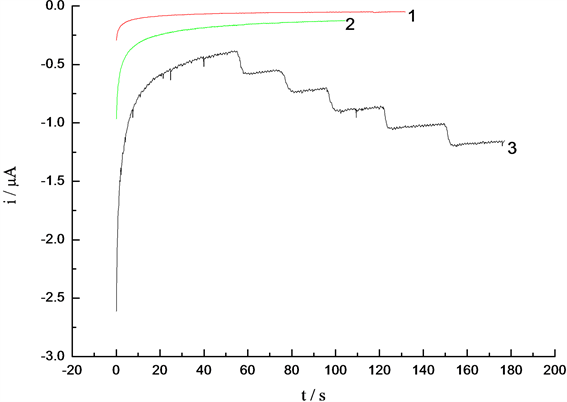Figure 3. Amperometric response of different electrodes for successive addition of glucose to 0.1 mol/L NaOH solution at 0.6 V; (1) Co/Ni modified CPE; (2) CPE; (3) Cu modified CPE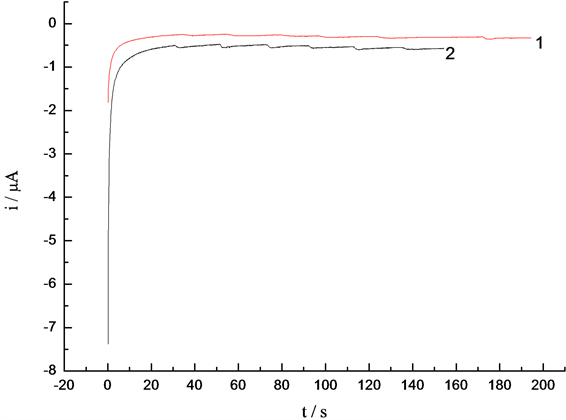Figure 4. Amperometric response of different electrodes for successive addition of glucose to 0.1 mol/L NaOH solution at 0.6 V; (1) deposition potential: −1 V; (2) deposition potential: −1.2 V

3.5. 电沉积时间的影响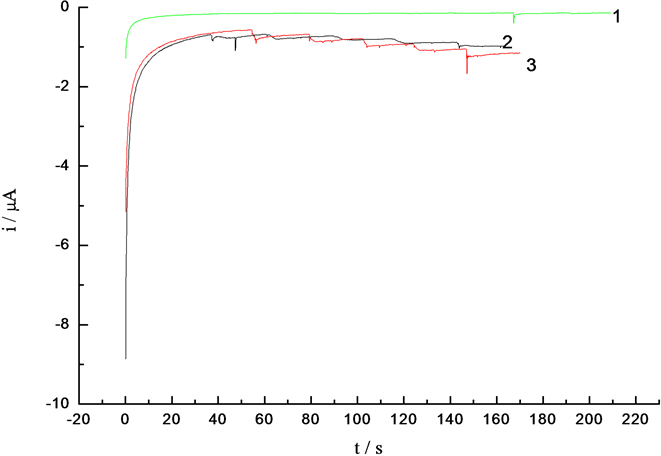Figure 5. Amperometric response of different electrodes for successive addition of glucose to 0.1 mol/L NaOH solution at 0.6 V; (1) deposition time: 20 s; (2) deposition time: 80 s; (3) deposition time: 40 s

3.6. 葡萄糖定量检测

3.6.1. 工作电位的影响

3.6.2. 线性范围与检出限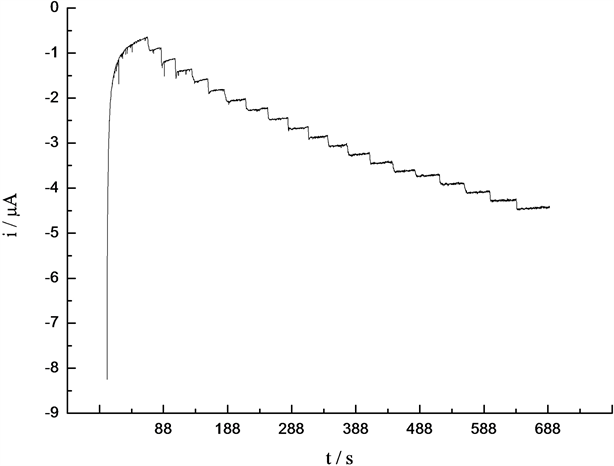Figure 6. Chronoamperogram of Glucose at Copper Modified Carbon Paste Electrode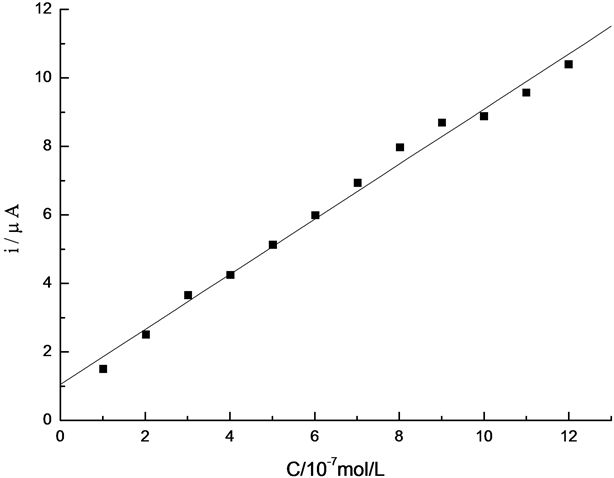Figure 7. Linear relationship between glucose concentration and current

3.7. 干扰实验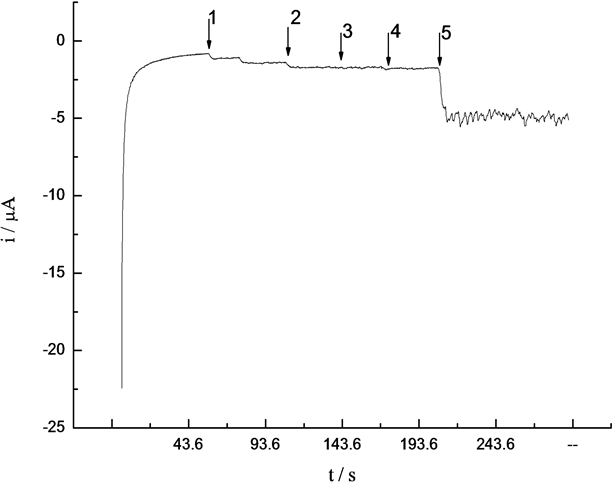Figure 8. Amperometric response of glucose in the presence of different interferents; 1 glucose; 2 urea 3; citric acid; 4 oxalate; 5 ascorbic acid

3.8. 电极的重现性、稳定性

3.9. 实际样品的测定Table 1. The determination of samples

4. 结论

  李彤, 姚子华, 丁良. 基于普鲁士蓝的葡萄糖氧化酶电极用于果汁饮料中葡萄糖测定[J]. 食品科学, 2005, 26(10): 187-190.  Yang, J., Jiang, L.C., Zhang, W.D. and Gunasekaran, S. (2010) A Highly Sensitive Non-Enzymatic Glucose Sensor Based on a Simple Two-Step Electrodeposition of Cupric Oxide (CuO) Nanoparticles onto Multi-Walled Carbon Nanotube Arrays. Talanta, 82, 25-33. https://doi.org/10.1016/j.talanta.2010.03.047  Xia, Y., Huang, W., Zheng, J.F., Niu, Z.J. and Li, Z.L. (2011) Nonenzymatic Amperometric Response of Glucose on a Nanoporous Gold Film Electrode Fabricated by a Rapid and Simple Electrochemical Method. Biosensors and Bioelectronics, 26, 3555-3561. https://doi.org/10.1016/j.bios.2011.01.044  Ahmad, M., Sun, H.Y., Hussain, M., Karim, S., Nisar, A. and Khan, M. (2015) Development of Silver Nanowires Based Highly Sensitive Amperometric Glucose Biosensor. Electroanalysis, 27, 1498-1506. https://doi.org/10.1002/elan.201400670  罗明荣, 王良良, 张亚静, 等. 基于聚多巴胺/铜微粒自组装多层膜的无酶葡萄糖传感器[J]. 分析化学研究报告, 2016, 44(6): 882-887.  Niu, X.H., Lan, M.B., Zhao, H.L. and Chen, C. (2013) Highly Sensitive and Selective Nonenzymatic Detection of Glucose Using Three-Dimensional Porous Nickel Nanostructures. Analytical Chemistry, 85, 3561-3569. https://doi.org/10.1021/ac3030976  Sun, Y.L., Yang, H.Y., Yu, X.H., Sun, Z.H., Meng, H.W. and Xu, X.H. (2015) A Novel Non-Enzymatic Amperometric Glucose Sensor Based on a Hollow Pt-Ni Alloy Nanotube Array Electrode with Enhanced Sensitivity. RSC Advances, 5, 70387-70397.  王艺兰, 包晓玉, 杨妍, 等. 电沉积Cu2O在无酶葡萄糖传感器中的应用[J]. 分析科学学报, 2016, 32(1): 71-74.  俞建国, 李建平. 高选择性的镍基无酶葡萄糖微传感器的研制及应用[J]. 分析化学研究报告, 2008, 36(9): 1201-1206.  潘芊秀, 程远征, 王江云, 等. 纳米铜/石墨烯-壳聚糖复合膜修饰电极及其对葡萄糖的直接测定[J]. 分析测试学报, 2017, 36(9): 1145-1149  Jin, J.J., Zheng, G.Y., Ge, Y.Y., Deng, S.G., Liu, W. and Hui, G.H. (2015) A Non-Enzyme Electrochemical Qualitative and Quantitative Analyzing Method for Glucose, D-Fructose, and Sucrose Utilizing Cu Foam Material. Electrochimica Acta, 153, 594-601. https://doi.org/10.1016/j.electacta.2014.11.194  王永华, 戚穗坚. 食品分析[M]. 北京: 中国轻工业出版社, 2017: 116-117.